# How to Add Units to Cells in Excel?

A unit is a measure of any value. For example, we measure mass in units of grams or kilobars. For each measure, there can be multiple units to measure it. We can't specify the measure for any value unless we have units. In general, we will try to represent every value in the same category with the same unit in Excel. Using the same units throughout the whole document helps to easily perform any calculations. We can add units to cells in Excel using two methods: using the format and using a formula.

## Adding Units to Cells Using "&"

Here we will first use the "&" operation to get the first result, then use the auto-fill handle to complete our task. Let's go over a simple procedure for adding units to cells in Excel.

Step 1

Let us consider an Excel sheet that contains the required data to add the units, similar to the below image.

Let us assume we want to add the units for mass; it will be kg.

Create a new column named "Mass" in Excel.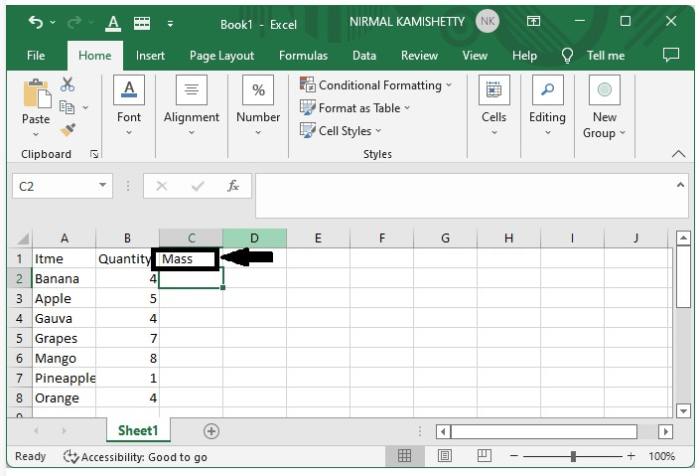Step 2

Now, in cell C2, enter the formula =B2&"kg" and press enter to get the first successful result.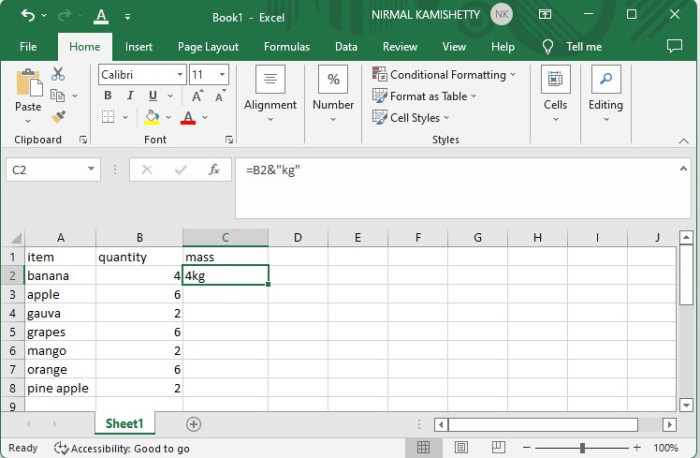We can get other results by dragging down from the right corner of the first result until all the results are acquired.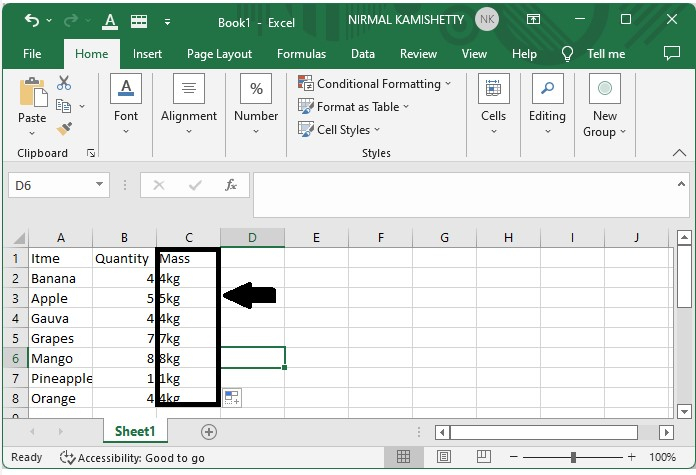## Adding Units to Cells Using Format

Here, we will first select the range of cells and format them to complete the task. Let's go over a simple procedure for adding units to cells in Excel using formatting.

Step 1

Let us consider the same data that we used in the above example.

Now enter the first value, select the whole column, and right-click and select Format Cells from the menu box.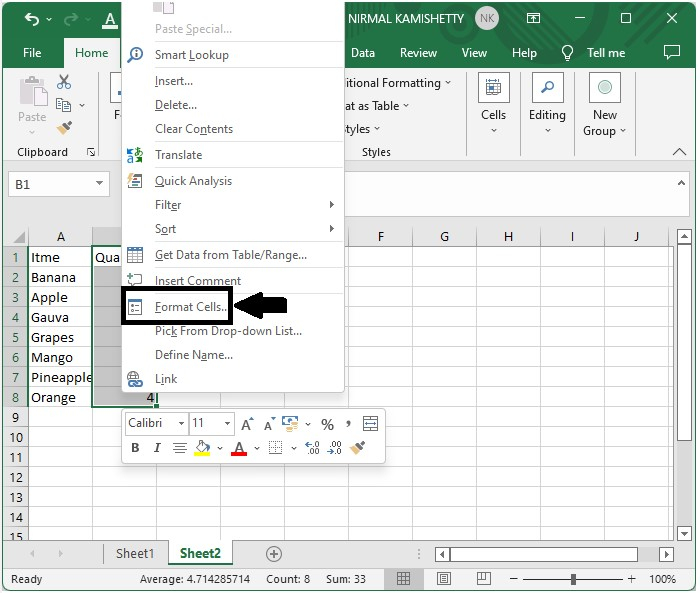Step 2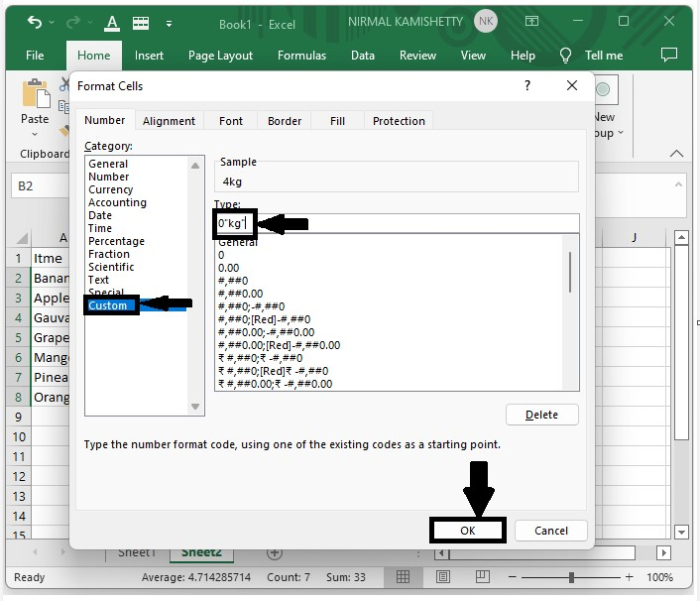Step 3

Now enter all the other values. The value will be converted to kg directly.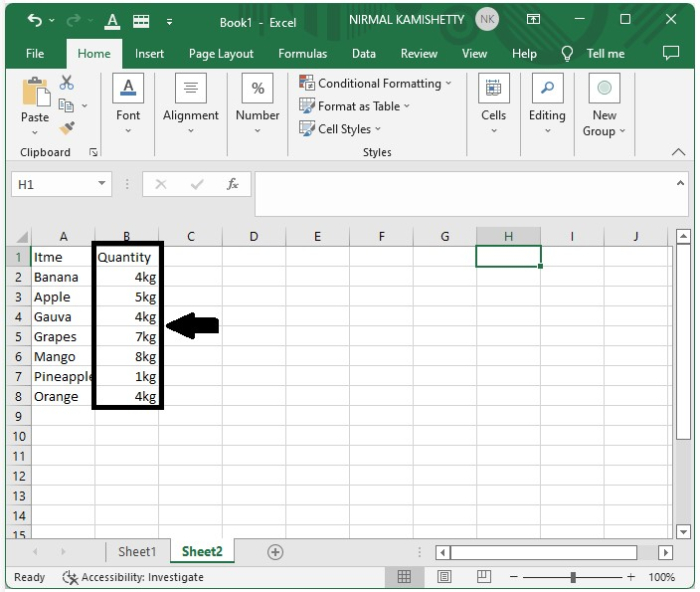## Conclusion

In this tutorial, we used a simple example to demonstrate how we can add units to cells in Excel to highlight a particular set of data.